Notes For Class 10 Science Physics Chapter 10 Light Reflection And Refraction Download In Pdf

Chapter 10 Light (Reflection and Refraction) notes download in pdf which has explanation of light reflection and refraction, light reflection and refraction class 10, light reflection and refraction class 10 notes, reflection and refraction of light

## Light (Reflection and Refraction) Notes

1. Ray of Light :

A line drawn in the direction of propagation of light is called a ray of light.

2. Beam of Light :

A group of rays of light emitted by a source of light is called a beam of light. A light beam is of three types.

(i) Parallel beam :
A group of light rays parallel to each other is known as parallel beam of light.

7. (ii) Divergent beam : A group of light rays spreading out from a source of light is called divergent beam of light.

(iii) Convergent beam : A group of light rays meeting at a point is called convergent beam 8. of light.

3. Reflection of Light :
There are some surfaces which have ability to send the light back in the same medium when light strikes it. This

9. phenomena of sending the light back in the same medium by a surface is called reflection of light
.

(i) The incident ray, the reflected ray and the normal at the point of incidence, all lie in a same plane.

(ii) The angle of incidence is always equal to the angle of reflection, ∠i = ∠r. 4. Image : When light rays meet or appear to meet after reflection from a mirror,then it is called an image.

1. Real Image :
It is a kind of image which is formed by actual intersection of light rays after reflection.

2. Virtual Image : It is a kind of image which is formed by producing the reflected rays backward after reflection.

5. Plane Mirror :
Plane mirror is a piece of glass whose one side is polished by using silver paint, which is covered by a coating of red paint to protect the silver layer.

6. Spherical Mirrors :
It is part of hollow glass sphere whose one surface is polished.

There are two types of spherical mirror.

(i) Concave Mirror :
It is a spherical mirror whose outer surface is polished and inner or concave side is reflecting surface.

(ii) Convex Mirror :
It is a spherical mirror whose inner is polished and outer side or convex side is the reflecting surface.

Principal Focus :

A point on the principal axis of a spherical mirror where the rays of light parallel to the principal axis meet or appear to meet after reflection from the mirror.

Focal Length :

The distance between the pole (P) and principal focus(F) of a spherical mirror is called the focal length of the mirror. It is denoted by f.

Uses of Concave Mirror :

(i) It is used as a shaving mirror because when it is placed close to the face, it forms a large image.

(ii) It is used in solar heating devices like solar cooker, because it converges Sun’s rays over a small area to produce high temperature.

(iii) It is used for security checking purposes. 10. Uses of

Convex Mirror :

(i) It is used as rear view mirror in automobiles because it gives erect image as well as diminished due to which Pt has wider field of view.

(ii) It is also used in street lights.

11. Mirror Formula :

It is a relation between distance of object, distance ofimage from the pole ofthe mirror and it’s focal length, i.e., relation between ‘u’, ‘v and It is given by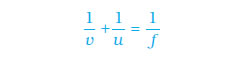12. Magnification :

It is defined as the ratio of height of image to the height of the object. It is denoted by letter m.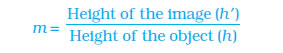### Laws of Refraction :

(i) The incident ray, the refracted ray and the normal at the point of incidence all lie in the same plane.

(ii) When a ray of light undergoes refraction then the ratio of sine of angle of incidence to the sine of angle of refraction is constant.

14. The Refractive Index :

The refractive index of medium 2 with respect to medium is given by the ratio of the speed of light in medium 1 and the speed of light in medium 2. This is usually represented by the symbol n21. This can be expressed in an equation form as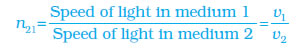15. Refraction by spherical lenses :

Lens is a transparent medium which is formed by joining two pieces of spherical glass. There are two types of lenses.

(i) Convex Lens :
It is a lens which is thicker at the centre and thinner at the edges.

(ii) Concave Lens : It is a lens which is thinner at the centre and thicker at the edges.

16. Terms related to a lens

Optical Centre of Lens :
It is the centre of the lens through which light can pass without any deviation

Principal Axis : It is the line passing through optical centre and is perpendicular to the line joining its edges.

Principal Focus :
It is a point on the principal axis where all light rays which are parallel to principal axis either converge or appear to diverge from, after refraction.

17. Lens formula :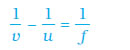18. Magnification :

Magnification, m = h2/h1

Ratio of height of image to the height of object.

It is also given by v/u i.e., Ratio of distance of image to the distance of object.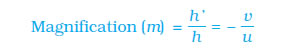19. Power of a lens :

A beam of light parallel to principal axis either gets converged or diverged after refraction by a lens. Some lenses converge the beam of light to a small extent and some lenses converge it to a large extent. This ability of lens to converge or diverge a beam of light is known as the power of lens.

Si unit of power of lens is dioptre :
One dioptre is the power of a lens whose focal length is 1 m.

Power of a combination of two or more lenses :

If two or more lenses are placed together to form a combined lens then the power of this combined lens is equal to the sum of the powers of individual lenses. P = P1 + P2 + P3 + ……

### NCERT Books Free Pdf Download for Class 5, 6, 7, 8, 9, 10 , 11, 12 Hindi and English Medium

 Mathematics Biology Psychology Chemistry English Economics Sociology Hindi Business Studies Geography Science Political Science Statistics Physics Accountancy